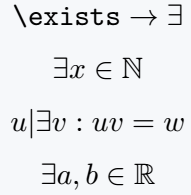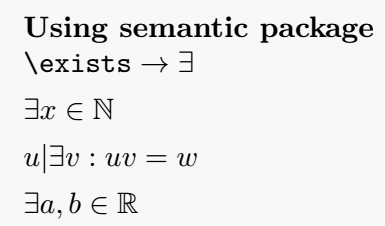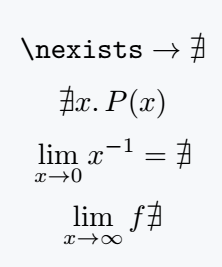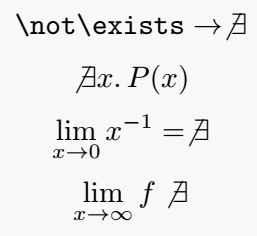# How do you write exist(∃) and not exist(∄) symbol in LaTeX?

In mathematical expressions, the Exist and does not exist symbols are required. In LaTeX, we will discuss the packages and commands required to write these two symbols in this tutorial.

## Exist symbol in LaTeX

LaTeX provides a default command for printing the Exist symbol in a document, which is \exists. This command must be used in latex math mode.

Symbol Exist
Type of symbol Mathematical Operators
Package No
Argument No
Latex command \exists
Example \exists → ∃

Below are some examples using this command.

\documentclass{article}
\usepackage{amssymb}
\begin{document}
% use amssymb package for \mathbb{}
$\verb|\exists|\rightarrow \exists$
$\exists x \in \mathbb{N}$
${u|\exists v: uv=w}$
$\exists a,b \in \mathbb{R}$
\end{document}

Output :LaTeX also has a package called semantic, which provides \exists commands. You can also print the Exist symbol in a LaTeX document using this package.

But using the default command and package, there is no difference in the output of the two methods. Take a look.

\documentclass{article}
\usepackage{amssymb}
\usepackage{semantic}
\begin{document}
\textbf{Using semantic package}\\
$\verb|\exists|\rightarrow \exists$\$4pt]  \exists x \in \mathbb{N} \\[4pt]  {u|\exists v: uv=w} \\[4pt]  \exists a,b \in \mathbb{R} \\ \end{document} Output :## Does not exist symbol in LaTeX You need a package to print the Does not exist symbol in a LaTeX document, which is amssymb. The amssymb package provides a \nexists command that allows you to print this symbol into a document. Symbol Does not exist Type of symbol Mathematical Operators Package amssymb Argument No Latex command \nexists Example \nexists → ∄ \documentclass{article} \usepackage{amssymb} \begin{document} \[ \verb|\nexists|\rightarrow \nexists$
$\nexists x.\,P(x)$
$\lim_{x \to 0}x^{-1}=\nexists$
$\lim_{x \to \infty}f\nexists$
\end{document}

Output :There is another alternative way to print the Does not exist symbol, if you use the \not\exists command, the symbol will be printed in a LaTeX document and you do not need to use any package.

But you will see some differences in the output of \nexists and \not\exists commands where the \nexists command gives better output.

For this, I would recommend using \nexists command.

\documentclass{article}
\begin{document}
$\verb|\not\exists|\rightarrow \not\exists$
$\not\exists x.\,P(x)$
$\lim_{x \to 0}x^{-1}=\not\exists$
$\lim_{x \to \infty}f\not\exists$
\end{document}

Output :#### Md Jidan Mondal

LaTeX expert with over 10 years of experience in document preparation and typesetting. Specializes in creating professional documents, reports, and presentations using LaTeX.